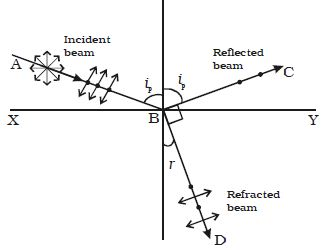# Describe Brewster’s Law

Sir David Brewster conducted a series of experiments with different reflectors and found a simple relation between the angle of polarization and the refractive index of the medium. It has been observed experimentally that the reflected and refracted rays are at right angles to each other when the light is incident at the polarizing angle.Fig: Polarization by reflection

From Figure, ip + 900 + r = 1800

r = 900 – iP

From Snell’s law, sin iP / sin r = μ

where μ is the refractive index of the medium (glass)

Substituting for r, we get

sin iP / sin (900 – iP) = μ;  sin iP / cos iP = μ

so,  tan iP = μ

The tangent of the polarizing angle is numerically equal to the refractive index of the medium.